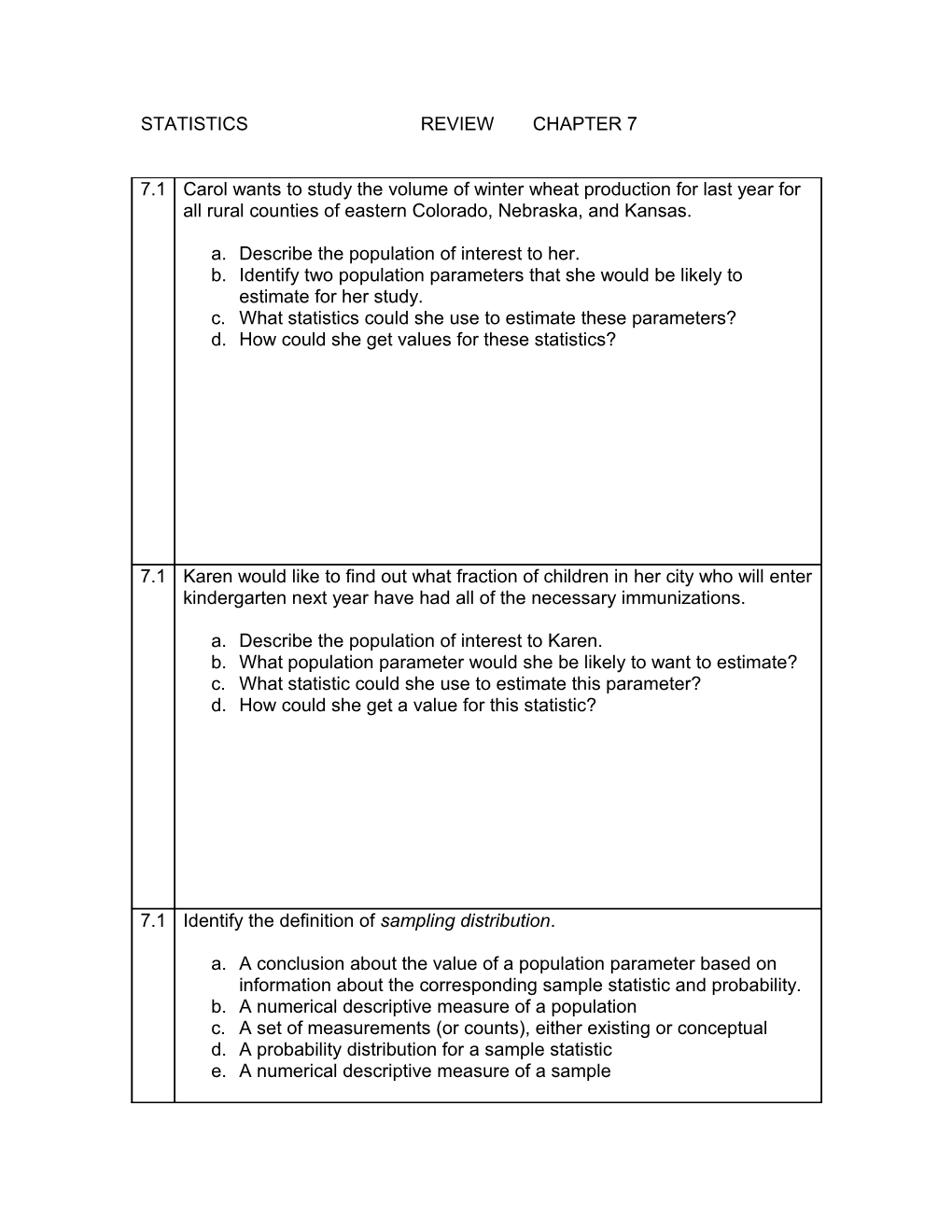# Describe the Population of Interest to HerSTATISTICSREVIEWCHAPTER 7

7.1 / Carol wants to study the volume of winter wheat production for last year for all rural counties of eastern Colorado, Nebraska, and Kansas.
1. Describe the population of interest to her.
2. Identify two population parameters that she would be likely to estimate for her study.
3. What statistics could she use to estimate these parameters?
4. How could she get values for these statistics?

7.1 / Karen would like to find out what fraction of children in her city who will enter kindergarten next year have had all of the necessary immunizations.
1. Describe the population of interest to Karen.
2. What population parameter would she be likely to want to estimate?
3. What statistic could she use to estimate this parameter?
4. How could she get a value for this statistic?

7.1 / Identify the definition of sampling distribution.
1. A conclusion about the value of a population parameter based on information about the corresponding sample statistic and probability.
2. A numerical descriptive measure of a population
3. A set of measurements (or counts), either existing or conceptual
4. A probability distribution for a sample statistic
5. A numerical descriptive measure of a sample

7.1 / Identify the definition of statistical inference.
1. A conclusion about the value of a population parameter based on information about the corresponding sample statistic and probability.
2. A numerical descriptive measure of a population
3. A set of measurements (or counts), either existing or conceptual
4. A probability distribution for a sample statistic
5. A numerical descriptive measure of a sample

7.1 / Identify an example of a population.
1. Seven cards chosen at random from a 52-card deck.
2. A week of television show watched by Americans as reported in a survey
3. The lengths of all trout in a lake
4. The automobiles bought by Americans polled in a telephone survey.
5. Registered Oklahoma voters who voted in a U.S. presidential election.

7.2 / The number of boxes of girl scout cookies sold by each of the girl scouts in a Midwestern city has a distribution which is approximately normal with mean μ=75 boxes and standard deviation σ=30 boxes.
1. Find the probability that a scout chosen at random sold between 60 and 90 boxes of cookies.
2. Find the probability that the sample mean number of boxes of cookies sold by a random sample of 36 scouts is between 60 and 90 boxes.
3. What difference do you observe in the two answers? Explain.

7.2 / Statistical Abstracts (117th ed) reports lengths of 78 shuttle flights. The distribution is somewhat asymmetric with mean μ=8.56 days and standard deviation σ=3.33 days.
1. Is the normal distribution a good approximation to the distribution of sample mean length of flights for random samples of size 4? Explain.
2. Find the probability that the sample mean for a random sample of 40 flights will be greater than 9 days.

7.2 / Let x be a random variable that represents white blood cell count per cubic milliliter of whole blood. Assume that x has a distribution that is approximately normal with mean μ=7500 and standard deviation σ=1750. Suppose that a doctor uses the average x-bar for three tests taken about a week apart. What is the probability of x-bar < 5000?
A 0.0764 B 0.4236 C 0.0035 D 0.0068 E 0.4932
7.3 / A candidate for city council is speaking to a breakfast group of 39 women about a law requiring a police permit to buy a gun. Assuming that 86% of American women favor such a law, what is the probability that three-fourths or more of the audience will support gun permits?
A 0.9972 B 0.8761 C 0.9864 D 0.8478 E 0.9179
7.3 / Courts sometimes make mistakes, but which do you believe is the worse mistake: convicting an innocent person or letting a guilty person go free? It turns out that about 60% of all Americans believe that convicting an innocent person is the worse mistake. Suppose you are taking a sociology class with 42 students enrolled. The question discussed today is: Do you agree with the statement that convicting an innocent person is worse than letting the guilty go free? What is the probability that the proportion of the class who agree is
1. at least one half?
2. at least two thirds?
3. no more than one third?
4. Is the normal approximation to the proportion p=r/n valid? Explain,

7.3 / A statistic course is taught each semester. The course always fills up to its maximum of 30 students. A P-Chart is to be made for the proportion of A’s given in the course each semester for the past 12 semesters. Each student is viewed as a binomial trial. Success means the student got an A, and failure is not getting an A. Since the class size is 30 each semester, the number of trials is n=30. Using information from the registrar’s office, the following data is obtained.
Sem / 1 / 2 / 3 / 4 / 5 / 6 / 7 / 8 / 9 / 10 / 11 / 12
R=# of A’s / 11 / 9 / 8 / 15 / 2 / 3 / 6 / 7 / 5 / 12 / 10 / 8
P=r/30 / .37 / .3 / .27 / .5 / .07 / .1 / .2 / .23 / .17 / .4 / .33 / .27
1. Make a P-Chart showing the proportion of A’s.
2. Determine whether the proportion of A’s is in statistical control. If it is not, specify which out-of-control signals are present.